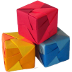# Sonobe Origami Polyhedra – Challenges

This page contains challenge questions about mathematical origami and links to other sources of questions. I know the answers to some of these questions. Some of the questions are open, however. This means that you could be the first person to ever work on them! I would love to hear from you by e-mail if you attempt any of these problems or would like some hints. I would be happy to post your answers on my web page or link to your solution elsewhere on the web.

### Polyhedra

Although all of the questions in this section are specific to Sonobe polyhedra, they can be adapted to polyhedra made from other origami modules.

• Using Sonobe units, can you build a stellated octahedron? A stellated icosahedron? (See the bottom of Stephan Lavavej's webpage for hints and pictures of these polyhedra. Stephan simply calls them "octahedron" and "icosahedron".)
• Can you use Sonobe units to make an unusual polyhedron?
• What is the smallest number of Sonobe units you need to make a polyhedron that has some volume (is not flat)? If you use just one module and insist that both flaps get tucked into different pockets, what shape do you end up with?
• How many different polyhedra can you make using six or fewer Sonobe units? Seven units? Eight?
• Can you find two different Sonobe polyhedra that fit together (like building blocks) to make a stellated octahedron? How many different ways can you do this? Are there two Sonobe polyhedra that fit together to make a standard-sized Sonobe cube?
• Can you construct a larger Sonobe polyhedron that looks like two or more small Sonobe polyhedra glued together? Is there a fundamental Sonobe polyhedron (or collection of polyhedra) that can be used to construct all larger Sonobe polyhedra?
• A Sonobe polyhedron is 3-colorable if there is a way to construct it using only three colors so that no module inserts into one that is the same color. Can you find a 3-coloring for a stellated octahedron? What about for a cube? For a real challenge, find a 3-coloring for a stellated icosahedron! Can you find any polyhedra that are not 3-colorable? What are the fewest number of colors needed to find a coloring for these polyhedra?
• Can any Sonobe polyhedra be 2-colored? Can you find any patterns that would help you predict which types of polyhedra can be 3-colored or 4-colored?
• Examine the symmetries of a Sonobe cube made from pieces of only one color. Does the cube have any reflection symmetries? Does the cube have rotational symmetries? How many of each does it have? If you take into account the ways the Sonobe pieces actually fit together, does the cube have any reflection symmetries? What about rotational symmetries? Now try taking coloring into account. Can you find a way of coloring the cube that destroys some of the symmetries but retains other symmetries? Try answering these questions for some other Sonobe polyhedra.
• How many Sonobe pieces are needed to make a stellated icosahedron? Can you figure out a way to find the answer without counting them all?